# Seaborn - How To Check Kernel Density Estimates¶

Kernel Density Estimation (KDE) is a way to estimate the probability density function of a continuous random variable. It is used for non-parametric analysis.

Setting the hist flag to False in distplot will yield the kernel density estimation plot.

Basic Information on KDE To understand kernel estimators we first need to understand histograms whose disadvantages provide the motivation for kernel estimators. When we construct a histogram, we need to consider the width of the bins ( equal sub-intervals in which the whole data interval is divided) and the endpoints of the bins (where each of the bins start). As a result, the problems with histograms are that they are not smooth, depend on the width of the bins and the endpoints of the bins. We can alleviate this problem by using kernel density estimators.

To remove the dependence on the endpoints of the bins, kernel estimators center a kernel function at each data point. And if we use a smooth kernel function for our building block, then we will have a smooth density estimate. This way we have eliminated two of the problems associated with histograms. The problem of bin-width still remains which is tackled using a technique discussed later on.

More formally, Kernel estimators smooth out the contribution of each observed data point over a local neighborhood of that data point. The contribution of data point x(i) to the estimate at some point x$^{\textrm{*}}$ depends on how apart x(i) and x$^{\textrm{*}}$ are. The extent of this contribution is dependent upon the shape of the kernel function adopted and the width (bandwidth) accorded to it. If we denote the kernel function as K and its bandwidth by h, the estimated density at any point x is

\begin{displaymath} \hat(x)=\frac{1}{n}\sum_{i=1}^{n}K\left(\frac{x-x(i)}{h}\right)\end{displaymath}

where $\int K(t)dt=1$to ensure that the estimates f(x) integrates to 1 and where the kernel function K is usually chosen to be a smooth unimodal function with a peak at 0.

In :
import pandas as pd
import seaborn as sb
from matplotlib import pyplot as plt
sb.distplot(df['petal_length'],hist=False)
plt.show()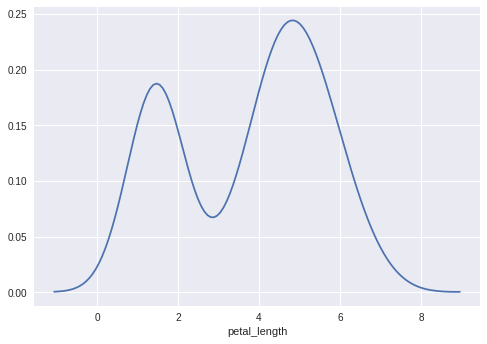In :
import pandas as pd
import seaborn as sb
from matplotlib import pyplot as plt
ax = sb.boxplot(x=df["petal_length"])
plt.show()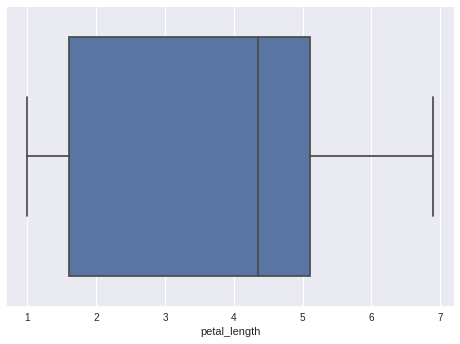In :
df.head()

Out:
sepal_length sepal_width petal_length petal_width species
0 5.1 3.5 1.4 0.2 setosa
1 4.9 3.0 1.4 0.2 setosa
2 4.7 3.2 1.3 0.2 setosa
3 4.6 3.1 1.5 0.2 setosa
4 5.0 3.6 1.4 0.2 setosa

Fitting Parametric Distribution

In :
import pandas as pd
import seaborn as sb
from matplotlib import pyplot as plt
sb.distplot(df['petal_length'])
plt.show()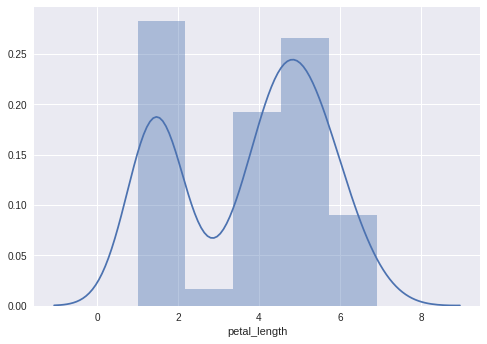## Points To Consider While Plotting Bivariate Distribution¶

Bivariate Distribution is used to determine the relation between two variables. This mainly deals with the relationship between two variables and how one variable is behaving with respect to the other.

The best way to analyze Bivariate Distribution in seaborn is by using the jointplot() function.

Jointplot creates a multi-panel figure that projects the bivariate relationship between two variables and also the univariate distribution of each variable on separate axes.

## Scatter Plot¶

Scatter plot is the most convenient way to visualize the distribution where each observation is represented in two-dimensional plot via x and y axis.

In :
import pandas as pd
import seaborn as sb
from matplotlib import pyplot as plt
sb.jointplot(x = 'petal_length',y = 'petal_width',data = df)
plt.show()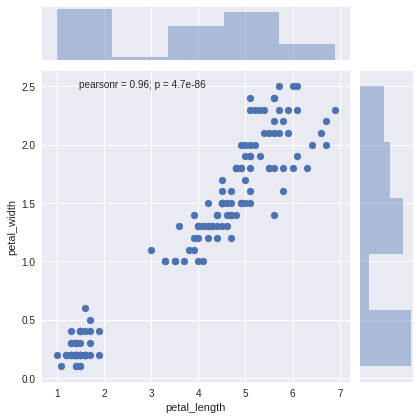The above figure shows the relationship between the petal_length and petal_width in the Iris data. A trend in the plot says that positive correlation exists between the variables under study.

## Hexbin Plot¶

Hexagonal Binning is used in bivariate data analysis when the data is sparse in density i.e., when the data is very scattered and difficult to analyze through scatterplots.

An addition parameter called ‘kind’ and value ‘hex’ plots the hexbin plot.

In :
import pandas as pd
import seaborn as sb
from matplotlib import pyplot as plt
sb.jointplot(x = 'petal_length',y = 'petal_width',data = df,kind = 'hex')
plt.show()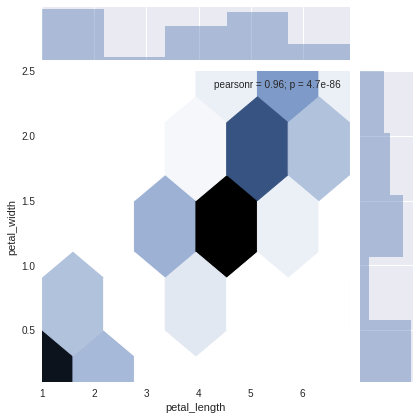## Kernel Density Estimation¶

Kernel Density Estimation is a non-parametric way to estimate the distribution of a variable. In seaborn, we can plot a kde using jointplot().

Pass value ‘kde’ to the parameter kind to plot kernel plot.

In :
import pandas as pd
import seaborn as sb
from matplotlib import pyplot as plt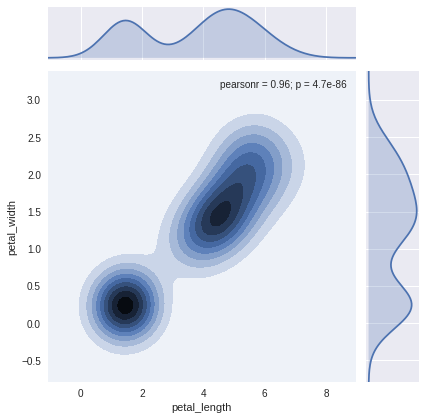Dolly Solanki